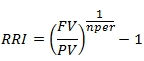# SQL Server RRI function

RRI
Updated: 4 Apr 2017

Use the scalar function RRI to calculate an equivalent interest rate for the growth of an investment. The formula is:FV = the future value of the investment PV = the present value of the investment nper = the number of periods.
Syntax
SELECT [wct].[RRI] (
<@Nper, float,>
,<@PV, float,>
,<@FV, float,>)
Arguments
 Input Name Description @Nper Number of periods. @Nper must be of type float or of a type that implicitly converts to float. @PV Present value of the investment. @PV must be of type float or of a type that implicitly converts to float. @FV Future value of the investment. @FV must of a type float or of a type that implicitly converts to float.
Return Type
float
Remarks
• If @PV is NULL then @PV = 0
• If @PV = 0 then NULL is returned
• If @FV is NULL then @FV = 0
• If @Nper is NULL then @Nper = 0
• If @Nper = 0 then NULL is returned
• Available in XLeratorDB / financial 2008 only
Example
SELECT
wct.RRI(
36      --@Nper
,1000    --@PV
,1100    --@FV
) as RRI

This produces the following result.Copyright 2008-2023 Westclintech LLC         Privacy Policy        Terms of Service